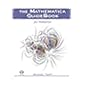Normal view

# The Mathematica GuideBook for Numerics [electronic resource] / by Michael Trott.

Material type:TextPublisher: New York, NY : Springer New York : Imprint: Springer, 2006Description: XXXVI, 1208 p. 789 illus. online resourceContent type: text Media type: computer Carrier type: online resourceISBN: 9780387288147Additional physical formats: Printed edition:: No titleDDC classification: 004 LOC classification: QA76.75-76.765Online resources: Click here to access online
Contents:
Introduction and Orientation -- I. Numerical Computations: Remarks -- Approximate Numbers -- Fitting and Interpolating Functions -- Compiled Programs -- Linear Algebra -- Fourier Transforms -- Numerical Functions and Their Options -- Sums and Products -- Integration -- Solution of Equations -- Minimization -- Solution of Differential Equations -- Two Applications -- Overview.-Exercises -- References -- II. Computation with Exact Numbers: Remarks -- Divisors and Multiples -- Number Theory Functions -- Combinatorial Functions -- Euler, Bernoulli, and Fibonacci Numbers -- Overview -- Exercises -- References -- Index.
Summary: Mathematica is today's most advanced technical computing system, featuring a rich programming environment, two-and three-dimensional graphics capabilities and hundreds of sophisticated, powerful programming and mathematical functions using state-of-the-art algorithms. Combined with a user-friendly interface and a complete mathematical typesetting system, Mathematica offers an intuitive, easy-to-handle environment of great power and utility. "The Mathematica GuideBook for Numerics" (text and code fully tailored for Mathematica 5.1) concentrates on Mathematica's numerical mathematics capabilities. The available types of arithmetic (machine, high-precision, and interval) are introduced, discussed, and put to use. Fundamental numerical operations, such as compiling programs, fast Fourier transforms, minimization, numerical solution of equations, ordinary/partial differential equations are analyzed in detail and are applied to a large number of examples in the main text and solutions to the exercises.
Tags from this library: No tags from this library for this title.Average rating: 0.0 (0 votes)
Item type Current location Collection Call number Status Date due Barcode Item holdseBook e-Library

Electronic Book@IST

EBook Available
Total holds: 0

Introduction and Orientation -- I. Numerical Computations: Remarks -- Approximate Numbers -- Fitting and Interpolating Functions -- Compiled Programs -- Linear Algebra -- Fourier Transforms -- Numerical Functions and Their Options -- Sums and Products -- Integration -- Solution of Equations -- Minimization -- Solution of Differential Equations -- Two Applications -- Overview.-Exercises -- References -- II. Computation with Exact Numbers: Remarks -- Divisors and Multiples -- Number Theory Functions -- Combinatorial Functions -- Euler, Bernoulli, and Fibonacci Numbers -- Overview -- Exercises -- References -- Index.

Mathematica is today's most advanced technical computing system, featuring a rich programming environment, two-and three-dimensional graphics capabilities and hundreds of sophisticated, powerful programming and mathematical functions using state-of-the-art algorithms. Combined with a user-friendly interface and a complete mathematical typesetting system, Mathematica offers an intuitive, easy-to-handle environment of great power and utility. "The Mathematica GuideBook for Numerics" (text and code fully tailored for Mathematica 5.1) concentrates on Mathematica's numerical mathematics capabilities. The available types of arithmetic (machine, high-precision, and interval) are introduced, discussed, and put to use. Fundamental numerical operations, such as compiling programs, fast Fourier transforms, minimization, numerical solution of equations, ordinary/partial differential equations are analyzed in detail and are applied to a large number of examples in the main text and solutions to the exercises.

There are no comments for this item.The Mathematica GuideBook for Numerics. by Trott, Michael. ©2005